# Smoothing Filters

 itk-DiscreteGaussianImage This filter computes the convolution of the input image with a Gaussian kernel. A one-dimensional Gaussian function is discretized on a convolution kernel. The size of the kernel is extended until ther are enough discrete points in the Gaussian to ensure that a user-provided maximum error is not exceeded. Usage: itk-DiscreteGaussianImage inputImageFile outputImageFile dimension [gaussian variance] [maximum kernel width] Below is an image and a filtered version with variance = 30, width = 0.5.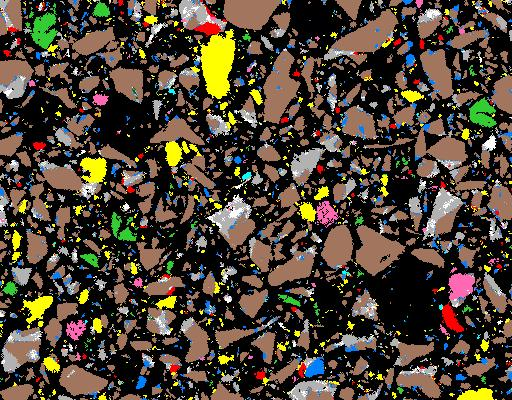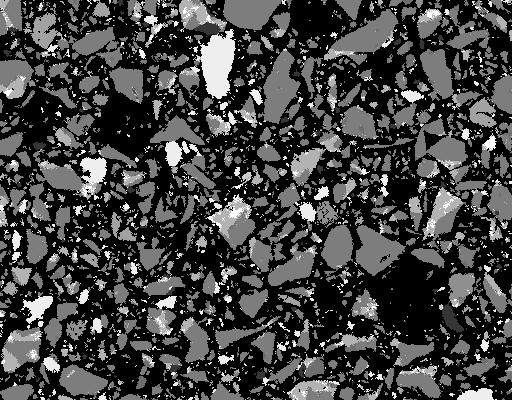itk-BinomialBlurImage This filter computes a nearest neighbor average along each dimension. The process is repeated a number of times, as specified by the user. A large number of iterations will approach the convolution with a Gaussian. Usage: itk-BinomialBlurImage inputImageFile outputImageFile dimension repetitions Below is shown an original image, and a image filtered 1, 2 and 5 times: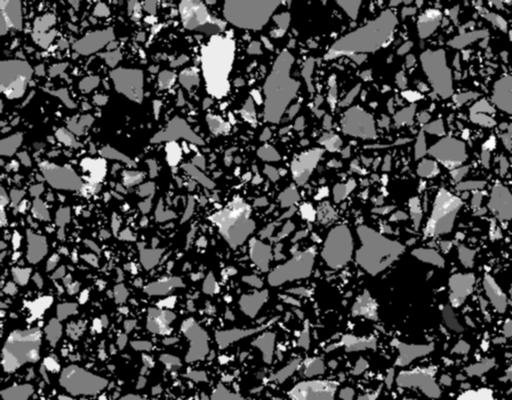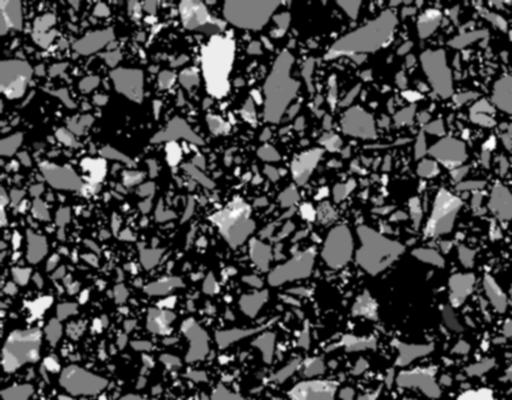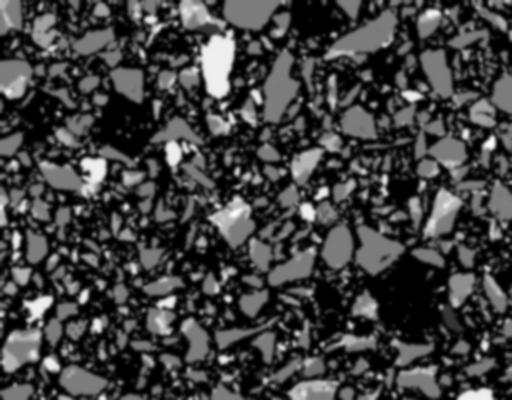itk-RecursiveGaussianImage This classical method of smoothing an image with a Gaussian kernel is slow when the standard deviation of the Gaussian is large. An approximation of convolution with the Gaussian and its derivatives is implemented using IIR^2 filters. Usage: itk-RecursiveGaussianImage inputImageFile outputImageFile dimension sigma Below is an original image, and the image filtered with sigma = 1,3,5: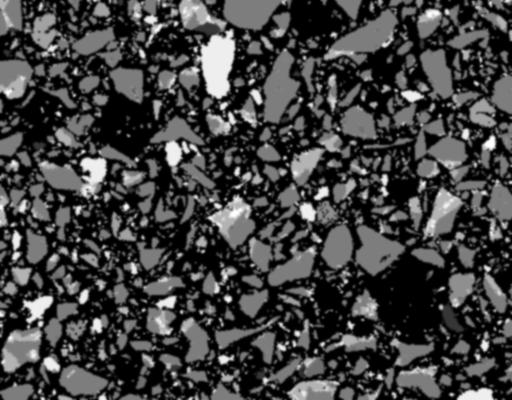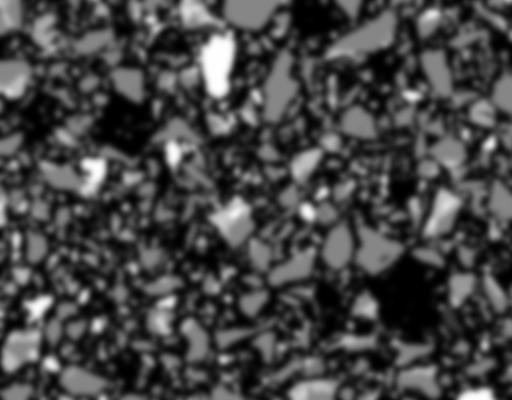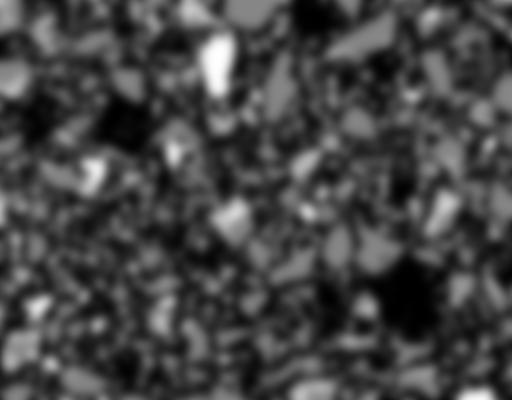itk-GradientAnisotropicDiffusionImage Anisotropic diffusion is a non-linear smoothing filter. It produces a Gaussian smoothed image, which is the solution to the heat equation, with a variable conductuce term to limit smoothing at edges. For this particular filter, the conductance term is a function of the gradient magnitude of the image at each point. This filter requires 3 parameters: the number of iterations to be performed, the time step, and the conductance parameter used in the computation of the level set evolution. Typical values are: number of iterations = 5, timestep = 0.25 for 2D, 0.125 for 3D. Usage: itk-GradientAnisotropicDiffusionImage inputImageFile outputImageFile dimension num_iterations timestep conductance Below is an original image, and an image with conductance = 1.0 and 5.0; timestep = 0.125, iterations = 5: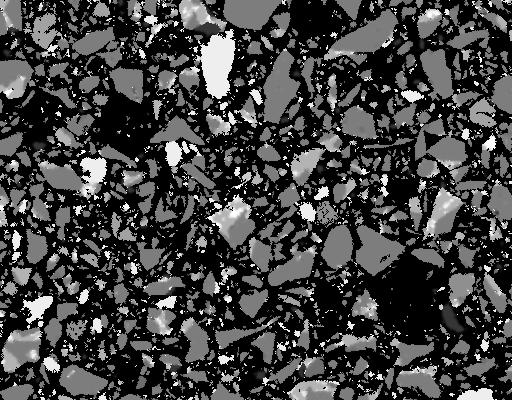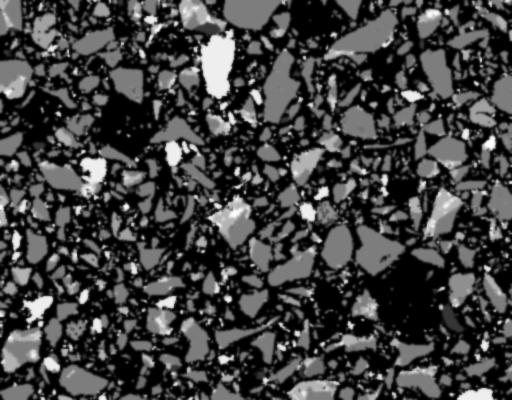itk-CurvatureAnisotropicDiffusionImage This filter performs anisotripic diffusion on an image using a modified curvature diffusion equation (MCDE). MCDE does not exhibit the edge enhancing properties of classic anisotropic diffusion. Usage: itk-CurvatureAnisotropicDiffusionImage inputImageFile outputImageFile dimension num_iterations timestep conductance Below is an original image, and an image with conductance = 1.0 and 5.0; timestep = 0.125, iterations = 5: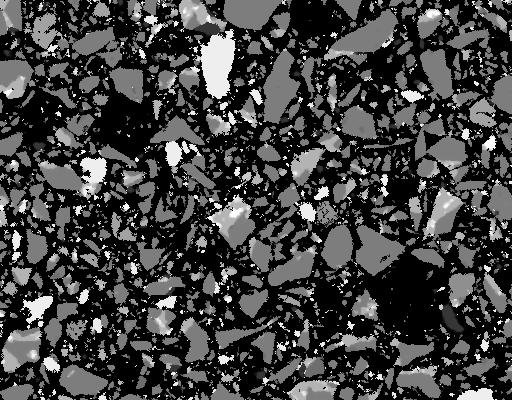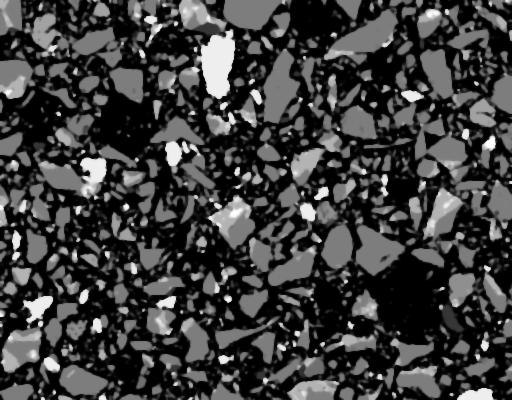itk-CurvatureFlowImage This filter performs edge-preserving smoothing similar to classical anisotropic diffusion. The filter uses a level set formation where the iso-intensity contours in an image are viewed as level sets, and pixels of a particular intensity form one level set. The level set function is then evolved under the control of a diffusion equation. Usage: itk-CurvatureFlowImage inputImageFile outputImageFile dimension num_iterations timestep Below is an original image, and an image with timestep = 0.125, iterations = 5: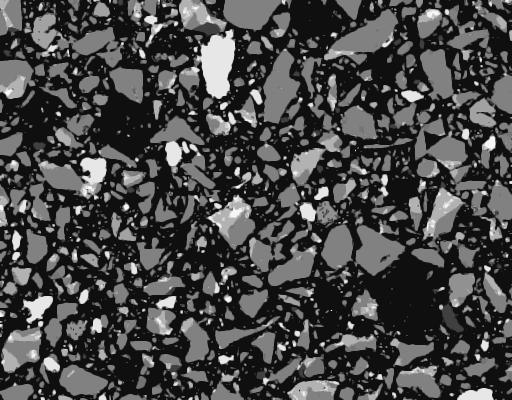itk-MinMaxCurvatureFlowImage This is a variant of the curvature flow filter above. Diffusion is turned on or off depending on the scale of the noise that one wants to remove. The scale is determined by a user defined neighborhood for each pixel, specified by a radius value. Usage: itk-MinMaxCurvatureFlowImage inputImageFile outputImageFile dimension num_iterations timestep radius Below is an original image, and an image with radius = 1, timestep = 0.125, iterations = 5 and 20: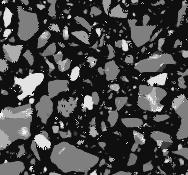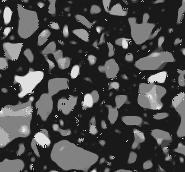itk-BilateralImage This filter performs smoothing using both domain and range neighborhoods. Two Gaussian kernels are used for the smoothing, one in the image domain and one in the image range. The result is an image that is smoothed in homogeneous regions yet has edges preserved. The results are similar to the anisotropic diffusion filter, but the implementation is non-iterative. Two parameters are required: A set of sigma values is used along each dimension in space. A second sigma value is used for image range smoothing. Usage: itk-BilateralImage inputImageFile outputImageFile dimension domainSigmas rangeSigma Below is an original image, and an image filtered with all sigma values set at 5.0: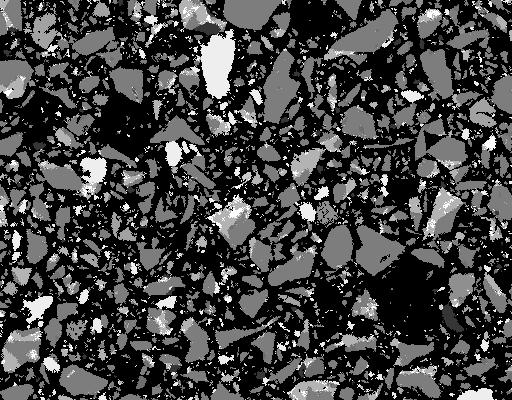itk-rgbGradientAnisotropicDiffusionImage This filter implements an N-dimensional version of the classic Perona-Malik anisotropic diffusion squation for vector-valued images. Perona and Malik introduced an alternative to linear-filtering, anisotropic diffusion. A gaussian smoothed image is a single time slice of the solution to the heat equation. Anisotropic diffusion uses a variable conductance term for this equation that depends on the structure of the image. The idea here is to limit the smoothing at edges, to keep the images sharp at the edges while smoothing the rest of the image. The vector anisotropic diffusion approach can be applied to color images. Each rgb component is diffused independently. This filter requires the same parameters as the other gradient anisotropic diffusion filters: Usage: itk-rgbGradientAnisotropicDiffusionImage inputImageFile outputImageFile dimension num_iterations timestep This images below are doubled in size to show the diffusion after 20 time steps of size=0.05: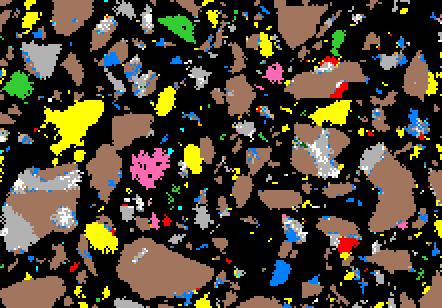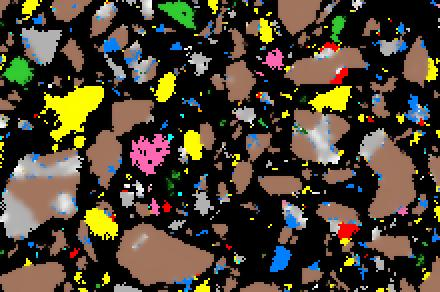itk-VectorCurvatureAnisotropicDiffusionImage This filter performs anisotripic diffusion on a vector image using a modified curvature diffusion equation. Vector components, in this case the rgb components, are diffused independently of one another. Usage: itk-VectorCurvatureAnisotropicDiffusionImage inputImageFile outputImageFile dimension num_iterations timestep The images below are doubled in size to show the diffusion after 20 time steps of size=0.05: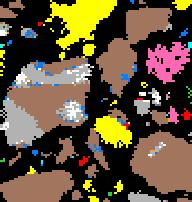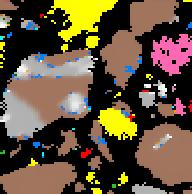Last updated November 18, 2005